#### You may also like### Have You Got It?

Can you explain the strategy for winning this game with any target?### Nim

Start with any number of counters in any number of piles. 2 players take it in turns to remove any number of counters from a single pile. The loser is the player who takes the last counter.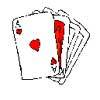### FEMTO

A new card game for two players.

# Dominoes

## Introduction

Here's a collection of games and puzzles using an ordinary set of dominoes. There are many more we could put in, including domino magic squares. We'll add to this collection in future features. Perhaps you would like to invent your own game; there are some ideas to build on here and NRICH will publish any good games sent in. You may find our Dominoes Environment useful too.

## A Domino Set

A set of dominoes usually consists of 28 rectangular dominoes, each having two squares with 0, 1, 2, 3, 4, 5, or 6 spots.

Every combination is represented. The value of the domino is the sum of the values of the two squares. Use a readymade set of dominoes or make one for yourself out of cardboard; then you can enjoy many different games and puzzles.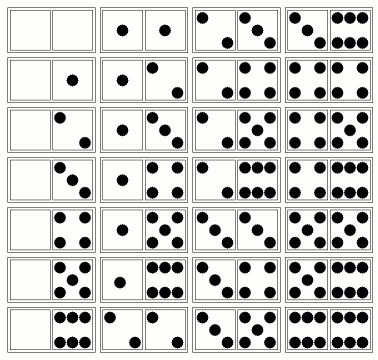## Basic rules for Dominoes

The basic rule in playing dominoes is that you make a chain of dominoes placing them end to end. In extending a chain, you always match one square of one of your dominoes to a square on the end of the chain. The exception to this is if you have a double domino - one with the same number on both squares. In this case, you can match both squares of the domino to one on the end of the chain, by placing the double domino perpendicularly across the end of the chain, like this: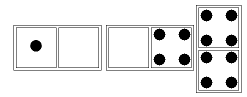This creates a branch in the chain, and you now count both squares on the double domino as ends of the chain which can be built upon. (Of course, if you prefer, you can just play the double domino in the usual way.)

The players all start with the same number of dominoes and you win if you are the first player to use up ALL your dominoes. If there are extra dominoes at the start they are placed face down as the 'pool'. Usually the player with the highest double starts. When it is your turn, you place one of your dominoes on one end of the chain and, if you cannot do so, then you have to pass, taking one domino from the pool if it is not empty.

## Rules for Fives and Threes

You play dominoes in the usual way but you score points when the dominoes at the ends of the chain add up to a multiple of 5 or a multiple of 3. Divide the total on the ends by 5 or 3 and add the answer to the player's score. If the end total is divisible by both 5 and 3 then you score both, so for the end total of 15 you score 8 points. You also score 10 points for being the first to finish, plus one point for every domino held by another player, but you might not be the winner even if you do finish first.

In the following example the (1,5) domino starts, scoring 2 points. Then the (0, 1) domino scores 1 point because the ends add up to 5. Then the (4,0) domino makes the ends add up to 9 so it scores 3 points. Finally the (5,6) domino makes the end total 10 scoring 2 points.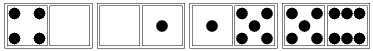In this example the (6,6) starts, scoring 4 points. Then the (6, 0) scores 4 points, then the (0,0) scores 4 points and finally, with the (0, 3) the end total is 15, scoring 8 points.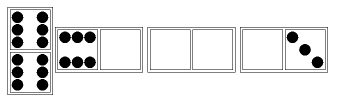## Domino aha!

If you make a chain with all 28 dominoes so that adjacent ends of dominoes match, and it has 5 spots at one end, how many spots will it have at the other end? You can work the answer out mentally and then check with the actual dominoes.

## Domino magic

Of course it isn't magic, just logic! There is a reason for everything in maths, sometimes hard to find but always there!

Remove and hide one domino (not a double) without telling anyone. People are unlikely to notice that one is missing. Ask a friend to make the usual chain and when he has put down three or four dominoes tell him the numbers that he will get at the ends when he has completed the chain. How do you do that? (Hint: apply the same logic as to Domino aha.)

## Windows

This is a challenge that you might like to take on by yourself or with a group of friends. In this diagram the four dominoes make a 'window' with one empty space. The spots on each side total nine. Can you make seven windows like this using all 28 dominoes so that each window has the same spot-sum for each side? One window need not have the same spot-sum as another.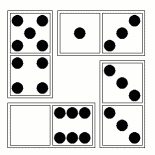Printable NRICH Roadshow resource.

Domino Multiplication

Here you have four dominoes laid out in the pattern of a multiplication sum. Can you make seven multiplication sums like this using all 28 dominoes? Again, like 'Windows' this organises the dominoes into seven sets of four.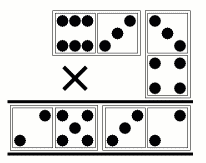Printable NRICH Roadshow resource.

## Domino Prime-squares

Here is a domino square made with six dominoes. The total number of spots on the sides are not the same, but they are ALL PRIME NUMBERS so we call it a 'prime-square'. The dominoes don't match end to end. Make some other domino prime-squares. Is it impossible to make a domino prime-square with the dominoes matching end to end? If so, why? What about squares with four dominoes, eight dominoes, or more?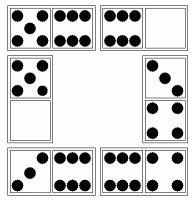## Create your own game

Once you have found seven windows, or seven multiplication sums, you have organised the dominoes into seven sets of four. Can you invent a game for 2 players where the object is to collect sets rather than to make chains? This could be a bit like the card game 'Rummy'. If you invent a good game do tell NRICH and we'll publish it on this page.